# The Biaugmented Pentagonal Prism

The biaugmented pentagonal prism (J53) is the 53rd Johnson solid. It has 12 vertices, 23 edges, and 13 polygons (8 triangles, 3 squares, and 2 pentagons).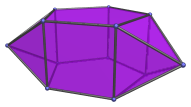It can be constructed augmenting a pentagonal prism with two square pyramids in non-adjacent positions. (Due to the dihedral angle of the augment with the adjacent square, adjacent augments would no longer be convex.) If only one augment is added, the augmented pentagonal prism (J52) is obtained instead.

## Projections

Here are some views of the biaugmented pentagonal prism from various angles:

Projection Description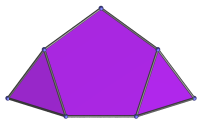Top view.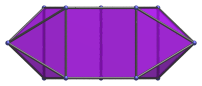Front view.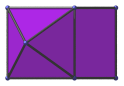Side view.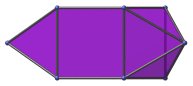72° rotated side view.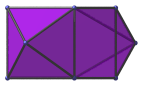144° rotated side view.

## Coordinates

The Cartesian coordinates of the biaugmented pentagonal prism, centered on the origin and with edge length 2, are:

• (0, √((10+2√5)/5), ±1)
• (±φ, √((5−√5)/10), ±1)
• (±1, −√((5+2√5)/5), ±1)
• (1/2)(±(φ2 + √(5+√5)), −((5−√5) + √(5+√5))/√10, 0)

where φ=(1+√5)/2 is the Golden Ratio.

Last updated 19 Jun 2018.